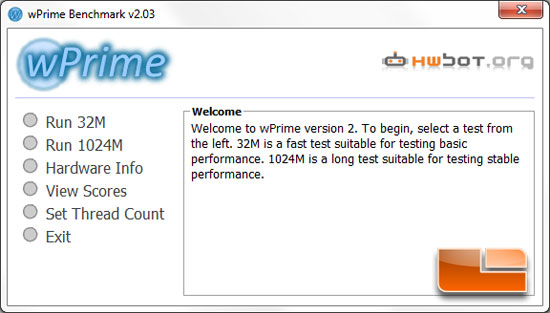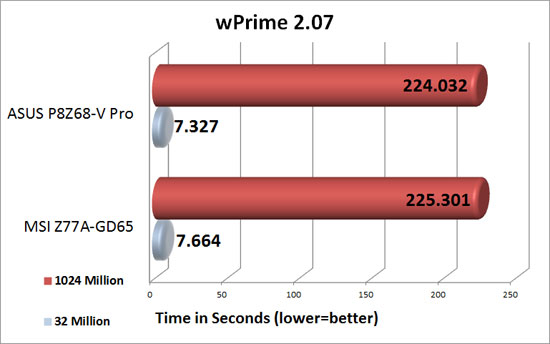# MSI Z77A-GD65 Motherboard Preview – Intel Z77 w/ Sandy Bridge

By

### wPrimewPrime is a leading multithreaded benchmark for x86 processors that tests your processor performance by calculating square roots with a recursive call of Newton’s method for estimating functions, with f(x)=x2-k, where k is the number we’re sqrting, until Sgn(f(x)/f'(x)) does not equal that of the previous iteration, starting with an estimation of k/2. It then uses an iterative calling of the estimation method a set amount of times to increase the accuracy of the results. It then confirms that n(k)2=k to ensure the calculation was correct. It repeats this for all numbers from 1 to the requested maximum.Benchmark Results: We were a little bit surprised to see as much of a difference as we did between the MSI Z77A-GD65 and the ASUS P8Z68-V Pro. In the 32 Million tests the ASUS P8Z68-V Pro was 4.4% faster than the MSI Z77A-GD65. Running the wPrime 1024 million test, the ASUS P8Z68-V Pro was only .56% faster than the MSI Z77A-GD65.